# Experiment of The Month

## Statistics of Marble Scattering

The MU Physics Department does not claim to have invented these labs. The origin of these labs is currently unknown to us. Our labs do not have written instructions. In keeping with this spirit, the description given here will be brief and general. The intent is that each performance of the lab will be unique; in each nature will reveal a slightly different face to the observer.

In the marble scattering laboratory, students measured the mean diameter of a population of marbles by scattering "shooter" marbles from target marbles. They used a statistical result for the uncertainty in their answer, which is derived here. The result is that the fractional uncertainty in the answer, for N repeated measurements is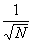. In the typical experiment, each group rolls 25 shooter marbles and counts the number of times that the shooter hits one of the target marbles. From this number of hits, they calculate a value, x, for the marble diameter. They repeat this experiment N times, calculating a marble diameter each time. Then the group calculates a mean diameter for their marbles, averaging over all N of their calculated marble diameters.

10 other groups do the same thing. To estimate the uncertainty in a single groups mean value for the diameter, we ask, "If the experiment is repeated, what is the range of diameters that we might expect?"

Since the experiment has been repeated by the other groups, we are able to answer this question. We calculate the standard deviation of the mean of the 10 diameters reported by the 10 groups. We call this error estimate the "standard deviation of the mean."

In the lab, it is verified that the standard deviation of the mean, divided by the mean value for the diameter (the "fractional deviation") is equal to. This month's article is devoted to a derivation of that result from basic statistical ideas of randomness.

The article is divided into two segments: The current page, which sets up the language and application, and Dr. Miziumski's page which shows the derivation.

We can display the data in an array, as shown, to help understand the analysis.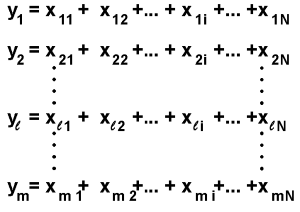In terms of the marble diameter experiment, each x in the array represents a single calculation of marble diameter, based on (for example) 25 rolls of the shooter marble. N is the number of those measurements (9, for example) that a single group used in their calculation of the mean diameter.

Still in the array, y is the sum of all N of the x values for one group. If we divide one of the y's by N, we have the mean diameter for that group. The number of groups in the laboratory is represented by m. This m is also the number of y's in the array. The mean value of the y's (called <y>) is calculated by summing all the y's and dividing by m. In principle, the mean of the y's is defined by letting m approach infinity. In practice, if there are 10groups in the laboratory, m=10.

With this value for <y>, we can calculate the deviations,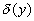and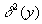from the mean for each y in the array. The average over them values foris the square of the standard deviation of the mean,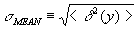.

It would be very useful to an individual group if there were a way to calculate the standard deviation of the mean based upon just their own data, without reference to other groups' results. Dr. Miziumski shows that the standard deviation of the mean is related in a simple way to the standard deviation of the x values.

This deviation of x's is calculated from the difference,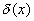between each individual x value and the average value of the N x's. The average over the N values of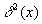is the square of the standard deviation of x (not the same as the standard deviation of the mean). We call this standard deviation,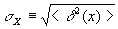.

The result is that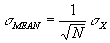This specific result leads to a much more general result: The fractional deviation of the mean is given by. To see this, we keep the number of target marbles (typically 12) and the number of shots per trial (e.g. 25) constant, and allow L, the width of the "Bowling alley" to become very large. See the figure for a definition of L.

When this happens, the number of hits (out of 25 rolls of a shooter marble) decreases. The calculated result for marble diameter stays the same, since it is determined by the product of number of hits times L.

Take this towards the extreme, so that most rolls of the shooter give no hits, giving a value of 0 for x. Approaching the extreme, the average value for x from any particular set (N trials of, for example, 25 rolls) approaches 0. The only other value that occurs with noticeable frequency is that from one hit for the 25 rolls of the shooter; x=L/25. Since the average value in a set of N trials is much closer to zero than to L/25, the x deviations in a given set are (essentially) the x values in the set.

This means that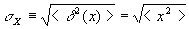. Thus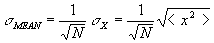Thus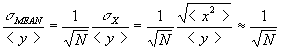## Result

Let x be as "Stochastic" or "Random" variable. This means that x occurs in "random sequences" x1, x2,..., xi,... of numbers with values belonging to a definite range (EG., +1 for heads, -1 for tails in a coin toss) each value appearing with a specific frequency (E.G., half heads, half tails.)

Partition a sequence of x into groups of N terms. Let the sum of the terms in the be yl . The object is to show that for long sequences, the averages (<>) of the squared deviations (d) of x and y are related by

<d2(y)>  =  N <d2(x)>  .

The mean of y is given by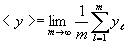. In a sequence of x, refer to the ith element (i = 1,2,...,N) of the l th group as xl , i . Then the corresponding element of the y sequence is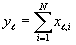. Notice that the mean of y is related to that of x by the expression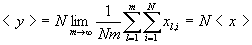because Nm is the total number of x values.

The deviation of the terms in the y-sequence from their mean can be expressed in terms of the corresponding deviation for x as follows:

dl (y) º yl -<y>

so that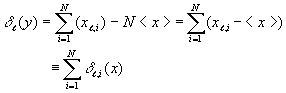Then the average of the squared deviation of y satisfies the equation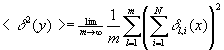so that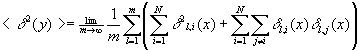or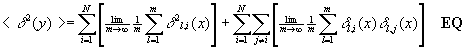.

Using the definition of independence sequences of random variables, easily justified using plausible arguments*, the value of the second bracketed term above is seen to be zero.

The bracketed expression in the surviving (first) term on the right hand side of EQ. is just <d2(x)> , so that the equation yields the predicted relationship,

<d2(y)>  =  N <d2(x)> .

* In the sum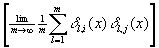sort the terms so that they are listed in order of increasing value for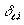. Since there are a large number of terms, many will be found for whichis the same, e.g.= .21. We collect those terms together. The constant value (e.g.= .21) multiplies the sum of many termsover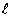.

But the sum of many's is zero. Repeating this, we see that the entire bracketed term is zero.

## Experiment Of The Month

View past labs experiments.